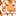# App Development

cancel
Showing results for
Did you mean:Partner

## Set Analyses with Nested If

Hi all'ya guru's

I'm trying to shorten the following set analyses statement:

if(vPeriod='YTD',
sum( {< yyyyFiscal={"\$(vFiscalYYYY)"}, pppFiscal={"<=\$(vFiscalPPP)"} >} ton ),
if(vPeriod='MTD',
sum( {< yyyyFiscal={"\$(vFiscalYYYY)"}, pppFiscal={"\$(vFiscalPPP)"} >} ton )
))

Have tried the following as an example, but not working:

sum({< yyyyFiscal={"\$(vFiscalYYYY)"}, pppFiscal ={"\$(=pick(match(\$(vPeriod),'YTD','MTD'),{"<=\$(vFiscalPPP)"},{"\$(vFiscalPPP)"}))" } >} ton )

Anyone have an idea of what the correct syntax should be or alternative function to use to bring it all under the same sum()

Labels (2)

• ### Set Analysis

1 Solution

Accepted SolutionsPartner

Usually, if the only difference in an expression is a single piece of text, you can leverage the variable to change the meaning.

In your example, the only difference is highlighted below in the two conditions:

if(vPeriod='YTD',
sum( {< yyyyFiscal={"\$(vFiscalYYYY)"}, pppFiscal={"<=\$(vFiscalPPP)"} >} ton ),
if(vPeriod='MTD',
sum( {< yyyyFiscal={"\$(vFiscalYYYY)"}, pppFiscal={"\$(vFiscalPPP)"} >} ton )
))

I would change the whole expression to:

sum( {< yyyyFiscal={"\$(vFiscalYYYY)"}, pppFiscal={"\$(vPeriodCondition)\$(vFiscalPPP)"} >} ton )

Where vPeriodCondition = '<=' for YTD or '' for MTD.

3 RepliesPartner

maybe this:

=sum( {< vPeriod = {'YTD'}, yyyyFiscal={"\$(vFiscalYYYY)"}, pppFiscal={"<=\$(vFiscalPPP)"} >} ton )
+
sum( {< vPeriod = {'MTD'}, yyyyFiscal={"\$(vFiscalYYYY)"}, pppFiscal={"\$(vFiscalPPP)"} >} ton )

replacing vPeriod with the field name.

I hope it can helps.Partner

Usually, if the only difference in an expression is a single piece of text, you can leverage the variable to change the meaning.

In your example, the only difference is highlighted below in the two conditions:

if(vPeriod='YTD',
sum( {< yyyyFiscal={"\$(vFiscalYYYY)"}, pppFiscal={"<=\$(vFiscalPPP)"} >} ton ),
if(vPeriod='MTD',
sum( {< yyyyFiscal={"\$(vFiscalYYYY)"}, pppFiscal={"\$(vFiscalPPP)"} >} ton )
))

I would change the whole expression to:

sum( {< yyyyFiscal={"\$(vFiscalYYYY)"}, pppFiscal={"\$(vPeriodCondition)\$(vFiscalPPP)"} >} ton )

Where vPeriodCondition = '<=' for YTD or '' for MTD.